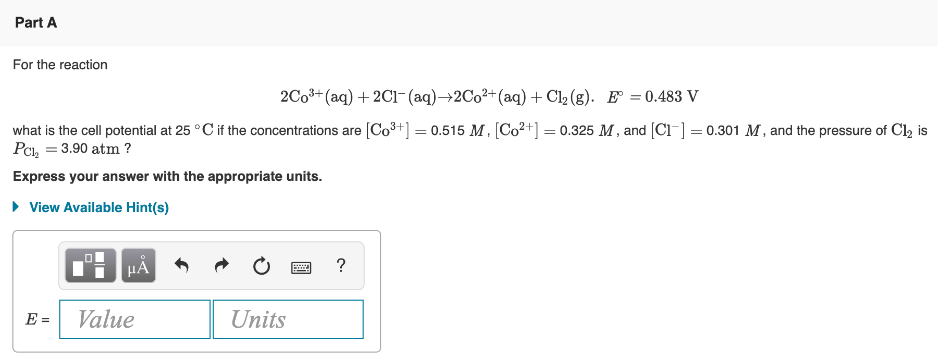# For the reaction 2Co^3+(aq) + 2Cl^-(aq) → 2Co^2+(aq) + Cl2(g). E° = 0.483 V what is the cell potential at 25 °C if the concentrations are [Co^3+] = 0.515 M, [Co^2+] = 0.325 M, and [Cl^-] = 0.301 M, and the pressure of Cl2 is PCl2 = 3.90 atm? Express your answer with the appropriate units.